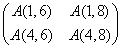5.1.1 定义数组

F90中变量的概念与F77的不同，它包含了两种变量，一种是标量，一种是数组。数组是科学和工程计算问题中常见的向量和矩阵的反映和概括。数组在FORTRAN程序中有着重要的意义，在批量大的情况下，如果不利用数组就失去了计算机的优越性。

a)    数组的描述

REAL        A(10,2,3)             ! 类型说明

DIMENSION   A(10,2,3)             ! DIMENSI0N语句

ALLOCATABLE B(:,:)                ! ALLOCATABLE语句

POINTER     C(:,:,:)              ! POINTER语句

REALDIMENSION(2,5):: D          ! 类型说明中的DIMENSION属性

REALALLOCATABLE::    E(:,:,:)   ! 类型说明中的ALLOCATABLE属性

REALPOINTER::        F(:,:)     ! 类型说明中的POINTER属性

b)    数组元素

1、数组名    -> 代表数组中所有元素；

2、数组元素  -> 代表数组中某一个元素；

3、数组片段  -> 代表数组中若干个元素，它们可以相连或分离。

B(2,2)=4.5; R7.O; C(INT(R)*2+1)=2.O !给元素B(2,2)C(15)赋值

A(1,2)=B(INT(C(15)),INT(SQRT(R)))     !元素A(1,2)和元素B(2,2)的值相同

c)    数组片段

d)    三元下标

A(1:5:2)=3.0  !把元素A(1)A(3)A(5)置为3.0

A(:5:2)=3.O   !把元素A(1)A(3)A(5)置为3.0，因为缺省下界为1

A(2::3)=3.O   !把元素A(2)A(5)A(8)置为3.0，因为上界缺省值为10

A(7:9)=3.0    !把元素A(7)A(8)A(9)置为3.0，因为缺省步长为1

A(:)=3.0      !A3.0相同，将A的所有元素置为3.0

A(1:4:3,6:8:2)=3.0e)    向量下标

INTEGER I(4),J(3)

I=(/5,3,8,2/)    !定义向量I

J=(/3,1,5/)      !定义向量J

A(I)=3.O         !设置元素A(5)A(3)A(8)A(2)的值

B(2,J)=3.O       !设置元素B(2,3)B(2,1)B(2,5)的值

a(b)a同类型，与b同形状，取值为(/0,3,2/)a(b)可以不是数组片段，而是更大的数组。如上面b(/2,3,2,3,2,3/)时，a(b)(/1,2,1,2,1,2/)

INTEGER :: bit(100)

bit的元素列为0001101100111...，则symbol(bit)是用{F,M}字符构成的100字节的字符型数组FFFMMFMMFFMMM...

INTEGER K(4)

K=(/3,1,1,2/)    !K矢量有重复值

A=5.0            !设置A的所有元素

B=A(3,K)         !数组片段B由下列元素组成：A(3,3),A(3,1),A(3,1),A(3,2)

5.1.2 数组类型

a)    显式形状(Explicit-shape)数组

b)    自动(Automatic)数组

DIMENSION A(N,5),B(10*N)

……

N=IFIX(R1)+IFIX(R2)

INTEGER A,B,LOWER

COMMON/BOUND/LOWER

……

INTEGER AUTO_ARRAY1(B)

INTEGER AUTO_ARRAY2(LOWER:B)

INTEGER AUTO_ARRAY3(20,B*A/2)

……

END SUBROUTINE

……

SUM1=THE_SUM(A1,10,35)

SUM2=THE_SUM(A2,3,56)

END

FUNCTION THE_SUM(A,M,N)

DIMENSION A(M,N)

SUMX=0.0

DO J=1,N

DO I=1,M

SUMX=SUMX+A(I,J)

END DO

END DO

THE_SUM=SUMX

END FUNCTION

L=9

M=5

CALL SUB(ARRAY,L,M)

END

SUBROUTINE SUB(X,I,J)

DIMENSION X(-I/2:I/2,J)

X(I/2,J)=999

J=1

I=2

END

a)    假定形状(Assumed-shape)数组

REAL A(:,:,:)

REAL X(4,7,9)

CALL ASSUMED(X)

SUBROUTINE SUB(M)

INTEGER M(:,1:,5:)

END SUBROUTINE

END INTERFACE

INTEGER L(20,5:25,10)

CALL SUB(L)

b)    假定大小(Assumed-size)数组

REAL A(2,2,*)

REAL X(7)

CALL ASSUME(X)

X(1)A(1,1,1)

X(2)A(2,1,1)

X(3)A(1,2,1)

X(4)A(2,2,1)

X(5)A(1,1,2)

X(6)A(2,1,2)

X(7)A(1,2,2)

c)    延迟形状(Deferred-shape)数组

INTEGER,ALLOCATABLE,TARGET:: K(:)

REAL,ALLOCATABLE,TARGET:: B(:,:),C(:)

ALLOCATE(A(2,3),C(5),B(SIZE(C),12))